TitleBeginning Algebra
Tutorial 13: Multiplication Property of EqualityLearning Objectives

 After completing this tutorial, you should be able to: Use the multiplication and division properties of equalities to solve linear equations.  Solve an equation using more than one property. Know how to express consecutive integers in terms of x, if the first integer is x. Know how to express even consecutive integers in terms of x, if the first even integer is x. Know how to express odd consecutive integers in terms of x, if the first odd integer is x.Introduction

 As mentioned in Tutorial 12: Addition Property of Equality, solving equations is getting into the heart of what algebra is about.  As we did in Tutorial 12,  we will be looking specifically at linear equations and their solutions.  We will start off slow and solve equations that use only the multiplication or division property of equality to make sure you have the individual concepts down.  Then we will pick up the pace and mix 'em up where you need to use several properties and steps to get the job done.  Equations can be used to help us solve a variety of problems. In later tutorials, we will put them to use to solve word problems.Tutorial

 The following definitions for equation, linear equation, solution and solution set can also be found in Tutorial 12: The Addition Property of Equality.

 Equation Two expressions set equal to each other.

 Linear Equation  in One Variable An equation that can be written in the form  ax + b = c where a, b, and c are constants.

 Note that the exponent (definition found in Tutorial 4: Introduction to Variable Expressions and Equations) on the variable of a linear equation is always 1.

 The following is an example of a linear equation: 3x - 4 = 5

 Solution A value, such that, when you replace the variable with it, it makes the equation true. (the left side comes out equal to the right side)

 Solution Set Set of all solutions

 Solving a Linear Equation  in General Get the variable you are solving for alone on one side and everything else on the other side using INVERSE operations.

 In Tutorial 12: The Addition Property of Equality, we showed you how to solve equations using the addition and subtraction properties of equality.  In this tutorial we will be looking at the multiplication and division properties.

 Multiplication and Division Properties of Equality If a = b, then a(c) = b(c) If a = b, then a/c = b/c where c is not equal to 0.

 In other words, if two expressions are equal to each other and you multiply or divide (except for 0) the exact same constant to both sides, the two sides will remain equal.  Note that multiplication and division are inverse operations of each other.  For example, if you have a number that is being multiplied that you need to move to the other side of the equation, then you would divide it from both sides of that equation.Example 1:  Solve the equation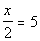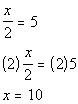*Inverse of div. by 2 is mult.  by 2

 If you put 10 back in for x in the original problem, you will see that 10 is the solution we are looking for.Example 2:   Solve the equation.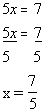*Inverse of mult. by 5 is div. by 5

 If you put 7/5 back in for x in the original problem, you will see that 7/5 is the solution we are looking for.Example 3:   Solve the equation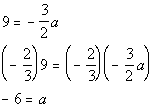*Inverse of mult. by -3/2 is div. by -3/2 (or mult. by reciprocal -2/3)

 If you put -6 back in for a in the original problem you will see that -6 is the solution we are looking for. Note that it doesn’t matter what side the variable is on.  -6 = a means the same thing as a = -6.

 The examples above and the ones from Tutorial 12: The Addition Property of Equality  were using only one property at a time to help you understand the different properties that we use to solve equations.  However, most times, we have to use several properties to get the job done.  The following is a strategy that you can use to help you solve linear equations that are a little bit more involved.

 Strategy for Solving a Linear Equation

 Note that your teacher or the book you are using may have worded these steps a little differently than I do, but it all boils down to the same concept -   get your variable on one side and everything else on the other using inverse operations.   Step 1: Simplify each side, if needed.   Step 2: Use Add./Sub. Properties to move the variable term to one side and all other terms to the other side.    Step 3: Use Mult./Div. Properties to remove any values that are in front of the variable.   Step 4:  Check your answer.   What it boils down to is that you want to get the variable you are solving for alone on one side and everything else on the other side using INVERSE operations.Example 4:  Solve the equation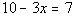.*Inverse of add. 10 is sub. 10     *Inverse of mult. by -3 is div. by -3

 Be careful going from line 4 to line 5.  Yes, there is a negative sign. But, the operation between the -3 and x is multiplication not subtraction.  So if you were to add 3 to both sides you would have ended up with -3x + 3 instead of the desired x.  If you put 1 back in for x in the original problem you will see that 1 is the solution we are looking for.Example 5:   Solve the equation.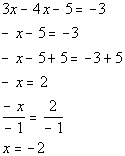*Simplify by combining like terms *Inverse of sub. 5 is add 5   *Inverse of mult. by -1 is div. by -1

 If you put -2 back in for x in the original problem you will see that -2 is the solution we are looking for.Example 6:   Solve the equation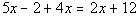.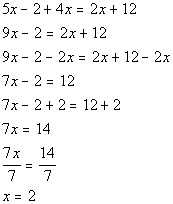*Simplify by combining like terms *Inverse of add 2x is sub. 2x *Inverse of sub. 2 is add 2 *Inverse of mult. by 7 is div. by 7

 If you put 2 back in for x in the original problem you will see that 2 is the solution we are looking for.

 Consecutive Integers

Consecutive integers are integers that follow one another in order.

 For example,  5, 6, and 7 are three consecutive integers.  If we let x represent the first integer, how would we represent the second consecutive integer in terms of x?  Well if we look at 5, 6, and 7 - note that 6 is one more than 5, the first integer.  In general, we could represent the second consecutive integer by x + 1.  And what about the third consecutive integer.  Well, note how 7 is 2 more than 5.  In general, we could represent the third consecutive integer as x + 2.

Consecutive EVEN integers are even integers that follow one another in order.

 For example, 4, 6, and 8 are three consecutive even integers.  If we let x represent the first EVEN integer, how would we represent the second consecutive even integer in terms of x?   Note that 6 is two more than 4, the first even integer.  In general, we could represent the second consecutive EVEN integer by x + 2.  And what about the third consecutive even integer?  Well, note how 8 is 4 more than 4.  In general, we could represent the third consecutive EVEN integer as x + 4.

Consecutive ODD integers are odd integers that follow one another in order.

 For example, 5, 7, and 9 are three consecutive odd integers.  If we let x represent the first ODD integer, how would we represent the second consecutive odd integer in terms of x?   Note that 7 is two more than 5, the first odd integer.  In general, we could represent the second consecutive ODD integer by x + 2. And what about the third consecutive odd integer?  Well, note how 9 is 4 more than 5.  In general, we could represent the third consecutive ODD integer as x + 4.   Note that a common misconception is that because we want an odd number that we should not be adding a 2 which is an even number.  Keep in mind that x is representing an ODD number and that the next odd number is 2 away, just like 7 is 2 away form 5, so we need to add 2 to the first odd number to get to the second consecutive odd number.Example 7:   Write an algebraic expression and simplify if possible.  If x represents the first of four consecutive integers, express the sum of the four integers in terms of x.

First of all, we need to have all four consecutive integers in terms of x

We can represent them the following way:

 x         = 1st integer x + 1   = 2nd consecutive integer x + 2   = 3rd consecutive integer x + 3   = 4th consecutive integer

Second we need to write it as a sum of the four integers and then simplify it: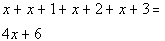*The sum of four cons. integers *Combine like termsExample 8:   Write an algebraic expression and simplify if possible.  If x represents the first of three odd consecutive integers, express the sum of the first and third integers in terms of x.

First of all, we need to have all three consecutive ODD integers in terms of x.

We can represent them the following way:

 x         = 1st odd integer x + 2   = 2nd consecutive odd integer x + 4   = 3rd consecutive odd integer

Second we need to write it as a sum of the first and third odd integers in terms of x and then simplify it: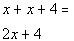*The sum of 1st and 3rd odd integers *Combine like termsPractice Problems

 These are practice problems to help bring you to the next level.  It will allow you to check and see if you have an understanding of these types of problems. Math works just like anything else, if you want to get good at it, then you need to practice it.  Even the best athletes and musicians had help along the way and lots of practice, practice, practice, to get good at their sport or instrument.  In fact there is no such thing as too much practice. To get the most out of these, you should work the problem out on your own and then check your answer by clicking on the link for the answer/discussion for that  problem.  At the link you will find the answer as well as any steps that went into finding that answer.Practice Problems 1a - 1d: Solve the given equation.

 1a.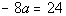(answer/discussion to 1a) 1b.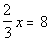(answer/discussion to 1b)

 1c.(answer/discussion to 1c) 1d.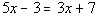(answer/discussion to 1d)Practice Problem 2a: Write an algebraic expression and simplify if possible.

 2a.  If x represents the first of three consecutive integers, express the sum of the three integers in terms of x. (answer/discussion to 2a)Need Extra Help on these Topics?

 Go to Get Help Outside the Classroom found in Tutorial 1: How to Succeed in a Math Class for some more suggestions.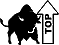Last revised on July 26, 2011 by Kim Seward.